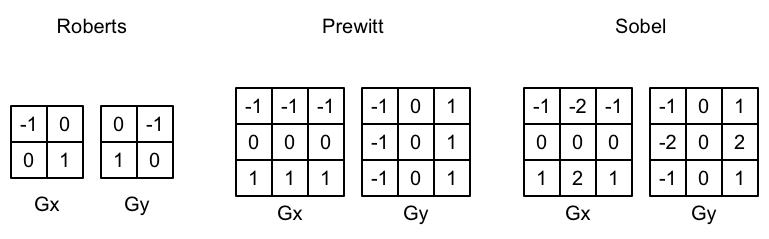## 后处理原理及过程

Unity提供了OnRenderImage()函数获取屏幕图像

MonoBehaviour.OnRenderImage (RenderTexture src, RenderTexture dest)

Blit函数获取屏幕图像后调用shader，将源图像src作为一张渲染纹理赋值给shader_MainTex

## 边缘检测(描边)

$$\frac{∂f(x,y)}{∂x}=\lim_{\epsilon->0}\frac{f(x+\epsilon,y)-f(x,y)}{\epsilon}$$

$$\frac{∂f(x,y)}{∂y}=\lim_{\epsilon->0}\frac{f(x,y+\epsilon)-f(x,y)}{\epsilon}$$Prewitt：$Gx = f(x+1) + 0 * f(x)-f(x-1)$，提取系数就是[-1, 0, 1]

Sobel就是prewitt的基础上，加强了对直线上像素的检测

## 高斯模糊和 Bloom 效果

$$G(x,y)=\frac{1}{2\pi\sigma^2}e^{-\frac{x^2+y^2}{2\sigma^2}}$$
$\mu$就是0，因为当前像素的原点距离为0，方差$\sigma$取标准方差1

n*n 的高斯核可以使用两个一维的高斯核替换

Bloom效果

luminance = 0.2125 * r + 0.7154 * g + 0.0721 * b，可以看出绿色对亮度的贡献最大，红色次之，蓝色最小